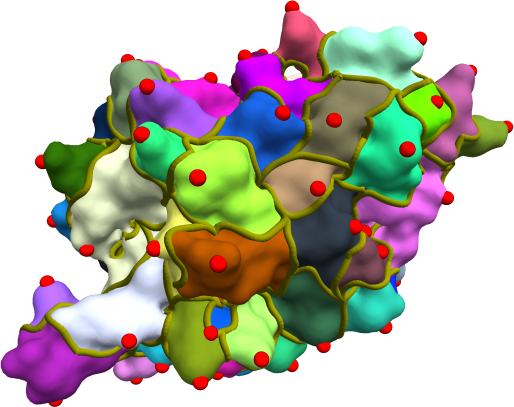#### Morse-Smale complexes: Computation and applications.

Nithin Shivashankar

#### Abstract

In recent decades, scientific data has become available in increasing sizes and precision. Therefore techniques to analyze and summarize the ever increasing datasets are of vital importance. A common form of scientific data, resulting from simulations as well as observational sciences, is in the form of scalar-valued function on domains of interest. The Morse-Smale complex is a topological data-structure used to analyze and summarize the gradient behavior of such scalar functions. This thesis deals with efficient parallel algorithms to compute the Morse-Smale complex as well as its application to datasets arising from cosmological sciences as well as structural biology.

The first part of the thesis discusses the contributions towards efficient com- putation of the Morse-Smale complex of scalar functions defined on two and three dimensional datasets. In two dimensions, parallel computation is made possible via a paralleizable discrete gradient computation algorithm. This algorithm is extended to work efficiently in three dimensions also. We also describe efficient algorithms that synergistically leverage modern GPUs and multi-core CPUs to traverse the gradient field needed for determining the structure and geometry of the Morse-Smale complex. We conclude this part with theoretical contributions pertaining to Morse-Smale complex simplification.

The second part of the thesis explores two applications of the Morse-Smale complex. The first is an application of the 3-dimensional hierarchical Morse- Smale complex to interactively explore the filamentary structure of the cosmic web. The second is an application of the Morse-Smale complex for analysis of shapes of molecular surfaces. Here, we employ the Morse-Smale complex to determine alignments between the surfaces of molecules having similar surface architecture.

[PDF]